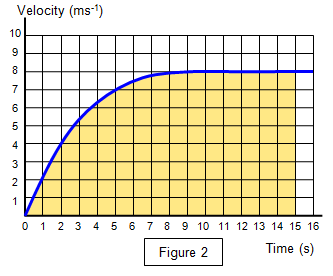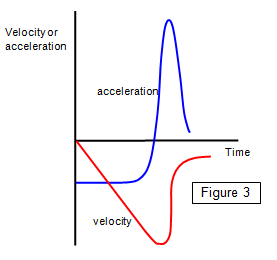# Acceleration-time graphs

Graphs of acceleration against time can be obtained from velocity-time graphs.

The slope of the line on a velocity-time graph at any point (dv/dt) gives the instantaneous acceleration.

The average acceleration is found by dividing the velocity change by the time taken.A velocity-time graph for an object moving with constant velocity is shown in Figure 1.

The velocity of the object changes constantly with time and so the acceleration of the object is constant and the acceleration-time graph for this motion is a straight horizontal line.

### Objects moving with varying accelerationThe graph in Figure 2 shows the change of velocity of a 100m sprinter. The acceleration at any point can be found by working out the gradient of the curve at that point.

This is looked at in more detail in:
Non-uniform accelerationOther complex motions can be analysed using the very useful material on the Multimedia Motion II CD available from Cambridge Science Media.

The graph in Figure 3 generated using the Cambridge Science Media package Multimedia Motion II shows the velocity-time (red line) and acceleration-time (blue line) graphs for a point on a man's knee when he jumps from a stool onto the ground.

A VERSION IN WORD IS AVAILABLE ON THE SCHOOLPHYSICS USB

© Keith Gibbs 2020